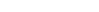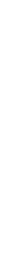# What is a confidence interval?

Describes the desired behavior of a confidence interval, that is, how to know whether a procedure produces a valid confidence interval.

Rona Axelrod email:rona.axelrod@fsw.edu , Daniel Kaplan https://dtkaplan.github.io
2020-06-16

Alternative document formats: Word & PDF

# Orientation

Statistics includes many forms and procedures for expressing uncertainty. A simple one is the assignment of a probability to a potential outcome. For instance, if we say the chance of rain tomorrow is 50%, we are expressing a large amount of uncertainty. In contrast, if the probability is specified as 10%, we are expressing much more certainty.

Another setting in which it’s helpful to express uncertainty involves a sample statistic such as the mean, standard deviation, median, correlation, regression slope, etc. After collecting a sample from the population, we simply calculate a sample statistic using the appropriate arithmetic; there is no uncertainty involved in that calculation. Where the uncertainty arises is in interpreting that calculated value. We would like to think that the calculated value can be taken as representative of the population from which the sample was collected. Indeed, we use phrases like “estimate the mean” to emphasize that we’re interested in the sample statistic only insofar as it is a good approximation to the population parameter.

The challenge is how to tell how well the sample statistic corresponds to the population parameter. This is a problem simple because we do not know the population parameter. Without this basic information about the population, we must be uncertain the extent to which the sample statistic corresponds to the population parameter.

Remarkably, it is possible to calculate from the sample alone and indicator of how well the sample statistic corresponds to the population parameter. The calculation produces a pair of numbers: the lower and upper bounds of what we call a confidence interval.

Often, statistics books devote many pages to how to calculate a confidence interval. We’re not going to do that here. The fact is, computers allow us to do the calculation just by asking for a confidence interval. We’re going to look at things a different way:

Suppose someone presented a computer program as a legitimate way to calculate a confidence interval? How would you know that the claim is correct?

The calculations for a legitimate confidence interval were designed by people, and usually there are different ways to calculate a legitimate interval on the same sample statistic.

But you can’t just make up your own interval and expect it to be legitimate. By analogy, you can put water in a bottle, shake it, and call it wine. But it won’t be legitimate wine. You can cut out cardboard wheels, paste them to a box, and call it a car. But it won’t be a legitimate car. This is common sense, since we are all very familiar with cars and wine. But there’s no common sense to identify an interval as a legitimate confidence interval.

In this activity, we’re going to present you with an interval and ask you to confirm that it is a legitimate confidence interval.

# Activity

1. Open the Little App on Confidence and T. (See footnote1). Pick the Source package to be Little Apps, pick the dataset to be NHANES2, pick the response variable to be systolic, and pick the explanatory variable to be select explanatory variable. Set the sample size to n-50 if it is not already there (click on the n= icon and pick n=50). The graph that will be displayed is very simple: A jittered point plot of the response variable from a random sample from the population. To keep the app simple, there is no explanatory variable. Just the response variable is shown with the horizontal axis used for jittering so that you can see the distribution of response variable.

Go into the apps control (the 3 parallel line icon). This opens a new window. Click the box next to show confidence interval. At the botton set the null hypothesis mu to be 119. On the graph you will see the population mean as a horizontal line at 119. Of course, ordinarily we don’t know the population mean, but this app uses the whole data set, typically many thousands of cases, as a stand-in for the population and if you use all the mean is 119.


There is also an interval shown. It was calculated using a standard technique for finding the confidence interval on the mean from the sample.

Chances are, the confidence interval on your display includes the population parameter. The probability of that being the case is the meaning of the *confidence level*, which by default is 95%.

* Since the default  confidence level is 95%, it's to be expected  that the vast majority of the time the confidence interval will include the population parameter. Press "New Sample" (the pair of dice icon) many times in  a row, noting after each press  whether the confidence interval generated by the new sample includes the population  parameter. Keep going until you encounter at least 2 intervals that don't cover the population parameter.

Did cover | ![](20mm-blank.png) |Did not cover

———-|————–|—————— ______ | | _________

1. Setting the confidence interval to 95% is an important statistical convention. In genuine statistical work, you should change it only for a good reason and make sure that the confidence level you are using is presented clearly in any report of your work. But for us here, 95% is inconvenient. The problem is that in addition to confirming that the confidence interval covers the population parameter the vast majority (95%) of the time, we also want to confirm that it does not cover the population parameter sometimes – about 5% of the time for a 95% confidence level.

• Back in the Apps control, set the confidence level to 50%. (This is just for the purpose of this demonstration. Remember, 95% is the convention.) This implies that, from sample to sample, the confidence interval will cover the population parameter 50% of the time and will not cover it the other 50% of the time. Pressing “new sample” 20 times and record how many times the confidence interval does and does not cover the population parameter.
Did coverDid not cover
______ _________
2. A legitimate process for constructing confidence intervals will create intervals that work regardless of the choice of the response variable.

• Try a variety of response variables in the NHANES2 data set and see if the confidence interval covers the population parameter with the right frequency, that is, about 50% for a 50% confidence level and 95% for a 95% level. To find the population mean for your variable, pick n=all, and then click on Stats in the top tool bar and read off the mean, then in the Apps contol set the null hypothesis nmu to that value.

Your answer:_________

3. A legitimate process for constructing confidence interval will create intervals that work regardless of the size of the sample. It’s hard to make such intervals for very small samples, but for sample sizes bigger than a couple of dozen, the established methods work pretty well.

• Try some different sample sizes, say n = 500 or 1000. Confirm that the confidence intervals cover the population parameter with the right frequency. For each of those sample sizes, set the confidence level to 50% and record the number of times out of 20 different samples that the confidence interval covers the population parameter.
Did coverDid not cover
______ _________
4. In order for confidence intervals to behave in the right way, that is, to cover the population parameter at a frequency specified by the confidence level, valid confidence intervals tend to change systematically with the sample size and with the confidence level.

1. Keeping one sample on the display by pressing the freeze the current plot icon (snowflake icon) change the confidence level. Then click the Compare button in the top tool bar. Now chance the confidence level and compare the length of the interval. State if the interval length became wider or narrower for each increae in confidence level.
Confidence levelInterval length
50%
80%
95%
99%
99.99%
1. Come up with a general statement about how the length of the confidence interval depends on the confidence level.

Your answer:1. Similarly, at a fixed confidence level, try several different sample sizes and consider the length of the confidence interval as being wider or narrower. Record this information and, again, come up with a general statement that describes well how the length of the confidence interval depends on the sample size.
Sample sizeInterval length
50
100
500
1000
5000

Version 0.3, 2020-08-14